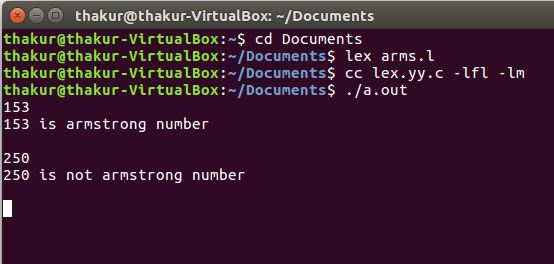Related Articles

# Lex program to check whether given number is armstrong number or not

• Last Updated : 09 May, 2019

Problem: Lex program to check whether given number is armstrong number or not.

Explanation:
Lex is a computer program that generates lexical analyzers and was written by Mike Lesk and Eric Schmidt. Lex reads an input stream specifying the lexical analyzer and outputs source code implementing the lexer in the C programming language.

Description:
An Armstrong number is a number that is the sum of its own digits each raised to the power of the number of digits. For example, 153 is an Armstrong number,

`(1^3) + (5^3) + (3^3) = 153 `

Examples:

```Input: 153
Output: 153 is a Armstrong number

Input: 250
Output: 250 is not a Armstrong number ```

Implementation:

 `/* Lex program to check whether given ``    ``- number is armstrong number or not */``%``{``/* Definition section */``#include ``#include ``    ``void` `check(``char``*);``    ``%``}`` ` `/* Rule Section */``% %``        ``[0 - 9]``    ``+ check(yytext);``% % `` ` `int` `main()``{``    ``/* yyin as pointer of File type */``    ``extern` `FILE``* yyin;``    ``yyin = ``fopen``(``"num"``, ``"r"``);`` ` `    ``// The function that starts the analysis``    ``yylex();`` ` `    ``return` `0;``}``void` `check(``char``* a)``{``    ``int` `len = ``strlen``(a), i, num = 0;``    ``for` `(i = 0; i < len; i++)``        ``num = num * 10 + (a[i] - ``'0'``);`` ` `    ``int` `x = 0, y = 0, temp = num;``    ``while` `(num > 0) {``        ``y = ``pow``((num % 10), len);``        ``x = x + y;``        ``num = num / 10;``    ``}`` ` `    ``if` `(x == temp)``        ``printf``(``"%d is armstrong number \n"``, temp);``    ``else``        ``printf``(``"%d is not armstrong number\n"``, temp);``}`

Output:Attention reader! Don’t stop learning now. Get hold of all the important CS Theory concepts for SDE interviews with the CS Theory Course at a student-friendly price and become industry ready.

My Personal Notes arrow_drop_up